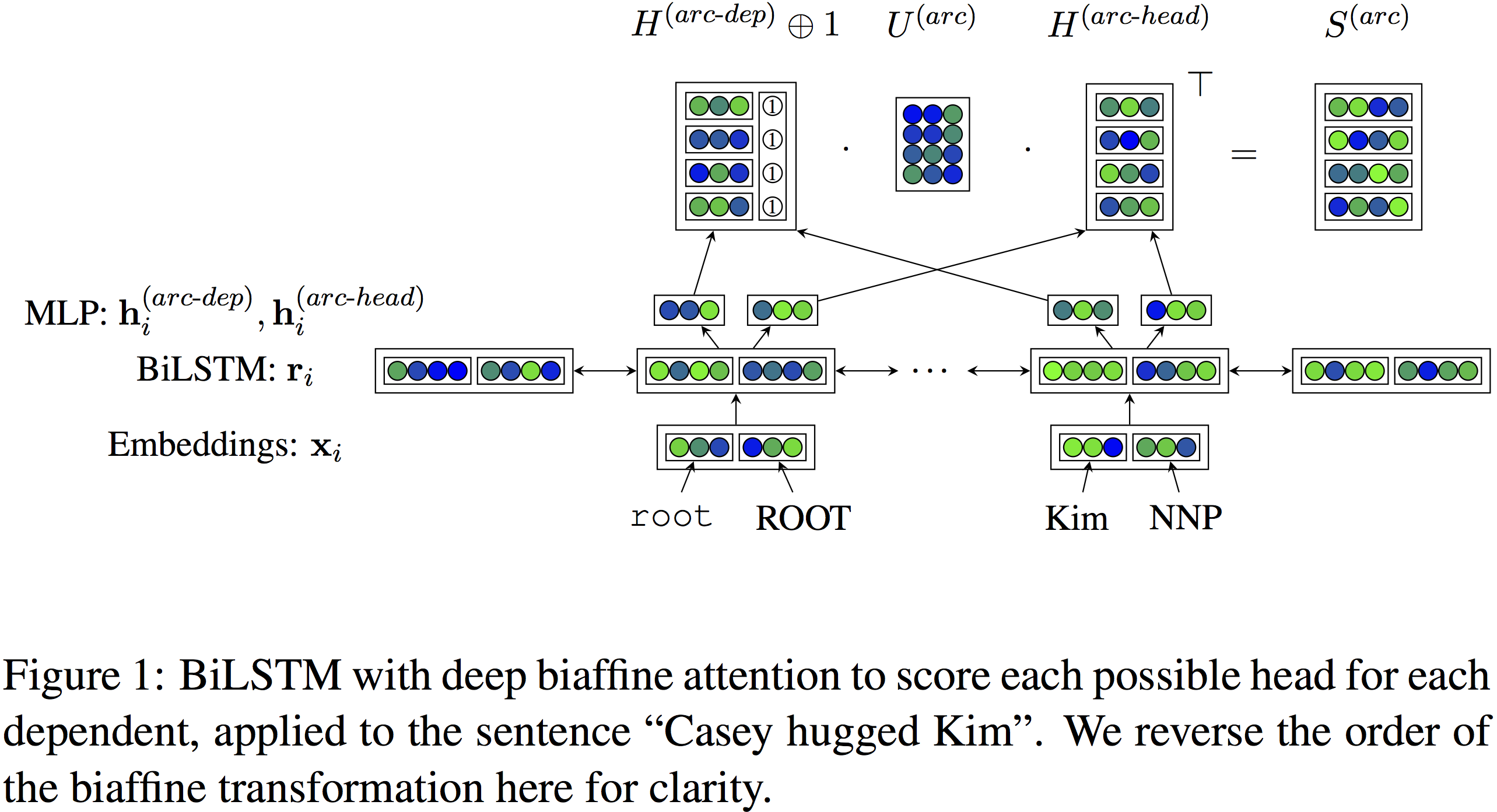# Deep Biaffine Attention for Neural Dependency Parsing

## arc得分

\begin{align} \label{tra-class}\mathbf{s}_i &= W\mathbf{r}_i + \mathbf{b} \end{align}

\begin{align} \label{arc-dep}\mathbf{h}_i^{(arc-dep)} &= \text{MLP}^{(arc-dep)}(\mathbf{r}_i)\\ \mathbf{h}_j^{(arc-head)} &= \text{MLP}^{(arc-head)}(\mathbf{r}_j) \end{align}

$$\label{arc-score}\mathbf{s}^{(arc)}_i = H^{(arc-head)}U^{(1)}\mathbf{h}^{(arc-dep)}_i+ H^{(arc-head)}\mathbf{u}^{(2)}$$

$$(d\times k) (k\times k)(k\times 1)+(d\times k)(k\times 1)=(d\times 1)$$

$$(d\times (k+1)) ((k+1)\times k)(k\times d)=(d\times d)$$## arc标签

\begin{align} \label{biaff-class}\mathbf{s}_i^{(label)} &= \mathbf{r}_{y_i}^\top \mathbf{U}^{(1)}\mathbf{r}_i + (\mathbf{r}_{y_i} \oplus \mathbf{r}_i)^\top U^{(2)} + \mathbf{b}\end{align}

$$\left( {\begin{array}{*{20}{c}} {\begin{array}{*{20}{c}} a \\ b \\ c \end{array}}&{\begin{array}{*{20}{c}} d \\ e \\ f \end{array}} \end{array}} \right)\left( {\begin{array}{*{20}{c}} {{x_1}} \\ {{x_2}} \end{array}} \right) = \left( {\begin{array}{*{20}{c}} {a{x_1} + d{x_2}} \\ {b{x_1} + e{x_2}} \\ {c{x_1} + f{x_2}} \end{array}} \right)$$

$$(1\times k)(m\times k \times k)(k\times 1)=(m\times 1 \times 1)$$

## 代码

# Linux
# Mac
pip install --upgrade \$TF_BINARY_URL

### 评论 2

1. #2

“同时进行d个token的运算，则上式的维度变化”这个地方跳跃太大了，虽然对应了figure中的公式的维度，但并不是上面公式的维度，而是d个s_i加了转置以后的S_arc公式的维度

风维月魄3年前 (2020-10-11)回复
2. #1

请问环境如何配置的呢？为什么在win7 pycharm 下面，总是报 cannot import name ‘Configurable’ 的错误呢？我已经
执行了 pip install Configurable 还望博主尽快回复。非常感谢

听风雨5年前 (2018-10-15)回复